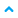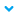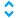# Expression evaluator

Calculate the result of an expression with integers, decimals, numeric variables, string variables for string comparisons, arithmetic and Boolean operators, or a set of functions.

## Properties

Property
Description
Expression
The expression to be calculated.
The expression can contain an unlimited number of correctly opened and closed parentheses. If the expression does not follow the correct syntax, the converter will not perform the calculation.
Mode
The direction of the dynamic link:
•Read from the source node to the parent.
•Write from the parent to the source node.
•Read from the source node to the parent and write from the parent to the source node.

## Source

One or more variables, identified by a placeholder with an index number in the following format {index}. The index number is progressive, must be an integer greater than zero {0}.
The placeholders can appear in any order in the expression.

## Valid literal values

• Boolean true and false.
• Integers.
• Decimals where the decimal separator must be a point.
• Hexadecimal numbers with the prefix "0x".
• Text such as a string delimited by double quotation marks.
TIP: Double quotation marks repeated twice (
""
) are interpreted as an escape sequence.

## Valid operators

• Arithmetical:
+
,
-
,
*
,
/
,
%
, and the unary operator
-
• Bitwise:
&
,
|
,
^
,
~
• Shift:
<<
,
>>
• Logics:
&&
,
||
,
!
• Unary casting of
(<data_type>)
type
• Comparison
<
,
<=
,
>
,
>=
,
==
,
!=
NOTE:
The comparison operators apply to:
• The numeric data
• DateTime data
• Duration data
The operators:
==
and
!=
also apply to String and LocalizedText data types.

## Data type conventions

• Literal integers are
Int32
.
• Decimal literals are
Double
.
• Numerical values are considered to be
Int32
.
• Literals true and false are interpreted as
Boolean
.
• The / operator always returns a
Double
value.
• The % operator generates an exception when there is division by modulo zero.
• The % operator accepts
Float
and
Double
operands.
• All functions return a
Double
value except for sign, which returns an
Int32
value.
• The unary casting operator accepts data types:
• bool, Boolean
• sbyte, SByte
• short, Int16
• int, Int32
• long, Int64
• byte, Byte
• ushort, UInt16
• uint, UInt32
• ulong, UInt64
• float, Single
• double, Double

## Available functions

The following mathematical functions are available:
Function
Calculated value
Example
max
Maximum of two values.
max ({0}, {1})
min
Minimum of two values.
min ({0}, {1})
avg
Average of given values.
avg ({0}, {1})
abs
Absolute value.
abs ({0})
trunc
Integer part of a decimal number.
trunc ({0})
ceil
Approximated value by excess.
ceil (({0} + {1})/2)
floor
Approximated value by defect.
floor (({0} + {1})/2)
round
Approximation to the nearest integer.
round (({0} + {1})/2)
sqrt
Square root.
sqrt ({1})
sign
0
if the argument value is greater than zero.
1
if the argument value is less than zero.
sign ({1})

## Output

The resulting value of the expression.

Provide Feedback